# Angular momentum

In physics, the angular momentum of an object rotating about some reference point is the measure of the extent to which the object will continue to rotate about that point unless acted upon by an external torque. In particular, if a point mass rotates about an axis, then the angular momentum with respect to a point on the axis is related to the mass of the object, its velocity, and its distance from the axis.

The concept of angular momentum is important in physics because it is a conserved quantity: a system's angular momentum stays constant unless an external torque acts on it. Torque is the rate at which angular momentum is transferred in or out of the system. When a rigid body rotates, its resistance to a change in its rotational motion is measured by its moment of inertia.

The conservation of angular momentum explains many phenomena in human activities and nature. For instance, it explains why an ice skater spins faster when drawing her arms close to her body, and slower when stretching her arms outward. It also explains why a compact star, such as a white dwarf, spins very fast, whereas the large star from which it was formed rotated much more slowly.

Knowledge of the angular momentum of an object also has important applications in engineering. For example, the kinetic energy stored in a rotating object such as a flywheel is proportional to the square of the angular momentum.

## Angular momentum in classical mechanicsRelationship between force (F), torque (τ), and momentum vectors (p and L) in a rotating system.

### Fundamental equation

The angular momentum of an object or particle that is moving around some origin (reference point) is defined by the following mathematical equation: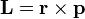$\mathbf{L}=\mathbf{r}\times\mathbf{p}$

where: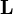$\mathbf{L}$ is the angular momentum of the object or particle,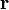$\mathbf{r}$ is the position of the object or particle expressed as a displacement vector from the origin,$\mathbf{p}$ is the linear momentum of the object or particle, and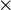$\times\,$ is the vector cross product.

The derived SI units for angular momentum are newtonmeterseconds, or N•m•s (kgm2s-1).

Because of the cross product, L is a vector perpendicular to both the radial vector r and the momentum vector p.

If a system consists of several particles moving around the same origin, the total angular momentum can be obtained by adding all the angular momenta of the constituent particles. Angular momentum can also be calculated by multiplying the square of the displacement r, the mass of the particle and the angular velocity.

### Angular momentum of group of particles

It is often convenient to consider the angular momentum of a collection of particles about their center of mass, because this simplifies the mathematics considerably. The angular momentum of a collection of particles is the sum of the angular momenta of each particle: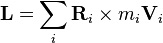$\mathbf{L}=\sum_i \mathbf{R}_i\times m_i \mathbf{V}_i$

where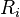$R_i$ is the distance of particle i from the reference point,$m_i$ is its mass, and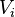$V_i$ is its velocity. The center of mass is defined by: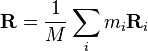$\mathbf{R}=\frac{1}{M}\sum_i m_i \mathbf{R}_i$

where$M$ is the total mass of all the particles.

If we define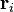$\mathbf{r}_i$ as the displacement of particle i from the center of mass, and$\mathbf{v}_i$ as the velocity of particle i with respect to the center of mass, then we have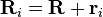$\mathbf{R}_i=\mathbf{R}+\mathbf{r}_i\,$   and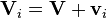$\mathbf{V}_i=\mathbf{V}+\mathbf{v}_i\,$

In this case, the total angular momentum is:$\mathbf{L}=\sum_i (\mathbf{R}+\mathbf{r}_i)\times m_i (\mathbf{V}+\mathbf{v}_i) = \left(\mathbf{R}\times M\mathbf{V}\right) + \left(\sum_i \mathbf{r}_i\times m_i \mathbf{v}_i\right)$

The first term is just the angular momentum of the center of mass. It is the same angular momentum one would obtain if there were just one particle of mass M moving at velocity V, located at the center of mass. The second term is the angular momentum that is the result of the particles spinning about their center of mass. The second term can be further simplified if the particles form a rigid body.

### Fixed axis of rotation

For many applications where one is concerned about rotation around a single axis, it is sufficient to discard the pseudovector nature of angular momentum and treat it like a scalar quantity. It is given a positive value for counterclockwise rotations, and a negative value for clockwise rotations. To do this, one takes the definition of the cross product and discards the unit vector, so that angular momentum becomes:$L = |\mathbf{r}||\mathbf{p}|\sin \theta_{r,p}$

where θr,p is the angle between r and p, measured from r to p. (One needs to make this distinction because without it, the sign of the cross product would be meaningless.) From the above, it is possible to reformulate the definition to either of the following: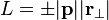$L = \pm|\mathbf{p}||\mathbf{r}_{\perp}|$

where r is called the perpendicular lever arm distance to p.

For an object with a fixed mass that is rotating about a fixed symmetry axis, the angular momentum is expressed as the product of the moment of inertia of the object and its angular velocity vector: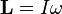$\mathbf{L}= I \mathbf{\omega}$

where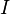$I\,$ is the moment of inertia of the object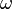$\mathbf{\omega}$ is the angular velocity.

### Conservation of angular momentum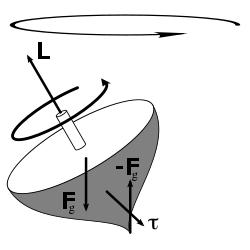The torque caused by the two opposing forces Fg and -Fg causes a change in the angular momentum L in the direction of that torque (since torque is the time derivative of angular momentum). This causes the top to move back upright.

In a closed system, angular momentum is constant. This conservation law follows mathematically from what is called the "continuous directional symmetry" of space—that is, no direction in space is any different from any other direction.

The change of angular momentum over time is called torque. Mathematically, it is expressed as the time derivative of angular momentum, as follows:$\tau = \frac{\mathrm{d}\mathbf{L}}{\mathrm{d}t} = \mathbf{r} \times \frac{\mathrm{d}\mathbf{p}}{\mathrm{d}t} = \mathbf{r} \times \mathbf{F}$

When the angular momentum is a constant (for a closed system), the mathematical expression for that is equivalent to the mathematical equation showing that the external torque acting on the system is zero. This equivalence may be written as follows: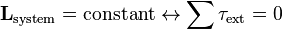$\mathbf{L}_{\mathrm{system}} = \mathrm{constant} \leftrightarrow \sum \tau_{\mathrm{ext}} = 0$

where$\tau_{ext}$ is any torque applied to the system of particles.

### Angular momentum of planetary orbits

For a planet in orbit, the angular momentum is distributed between the spin of the planet itself and the angular momentum of its orbit: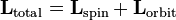$\mathbf{L}_{\mathrm{total}} = \mathbf{L}_{\mathrm{spin}} + \mathbf{L}_{\mathrm{orbit}}$;

If a planet appears to rotate slower than expected, astronomers suspect that the planet is accompanied by a satellite, because the total angular momentum is shared between the planet and its satellite in order to be conserved.

## Usefulness

The conservation of angular momentum is used extensively in analyzing what is called central force motion. If the net force on some body is always directed toward a fixed point, the center, then there is no torque on the body with respect to the center, and the angular momentum of the body about the center is constant. Constant angular momentum is extremely useful when dealing with the orbits of planets and satellites. This concept was also used for the Bohr model of the atom.

The conservation of angular momentum explains the angular acceleration of an ice skater as she brings her arms and legs close to the vertical axis of rotation (or close to her body). By bringing part of her body mass closer to the axis, she decreases her body's moment of inertia. Because angular momentum is constant in the absence of external torques, the angular velocity (rotational speed) of the skater has to increase.

The same phenomenon explains the extremely fast spin of compact stars (like white dwarfs and neutron stars) and black holes, when they are formed out of much larger and slower rotating stars. (Decreasing the size of an object 104 times results in increasing its angular velocity by a factor of 108).

## Angular momentum in quantum mechanics

To explain the behavior of subatomic particles, the theory of quantum mechanics indicates that the angular momentum of a particle is "quantized." In other words, the angular momentum of a subatomic particle does not vary continuously, but it changes in "quantum leaps" between certain allowed values. When a subatomic particle is moving through space, its angular momentum due to this motion is always a whole-number multiple of a constant denoted as$\hbar$ ("h-bar").

Experiments show that most subatomic particles also have a permanent, built-in angular momentum that is not due to their motion through space. This "spin" angular momentum comes in units of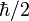$\hbar/2$. For example, an electron has a spin angular momentum of$\hbar/2$.

### Basic definition

As noted above, the classical definition of angular momentum can be written as:$\mathbf{L}=\mathbf{r}\times\mathbf{p}$

The value of angular momentum depends on six numbers: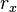$r_x$,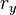$r_y$,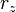$r_z$,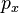$p_x$,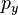$p_y$, and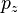$p_z$.

When dealing with particles on the subatomic scale, the Heisenberg uncertainty principle tells us that it is not possible for all six of these numbers to be measured simultaneously with arbitrary precision. Therefore, there are limits to what can be known or measured about a particle's angular momentum. It turns out that the best that one can do is to simultaneously measure both the angular momentum vector's magnitude and its component along one axis.

Mathematically, angular momentum in quantum mechanics is defined in the same way as momentum—not as a quantity but as an operator on the wave function:$\mathbf{L}=\mathbf{r}\times\mathbf{p}$

where r and p are the position and momentum operators respectively. In particular, for a single particle with no electric charge and no spin, the angular momentum operator can be written in the position basis as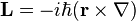$\mathbf{L}=-i\hbar(\mathbf{r}\times\nabla)$

where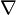$\nabla$ is the gradient operator, read as "del," "grad," or "nabla." This is a commonly encountered form of the angular momentum operator, though not the most general one.